# C++ Program to Perform Addition, Subtraction, Multiplication and Division

In this tutorial you will learn about the C++ Program to Perform Addition, Subtraction, Multiplication and Division and its application with practical example.

## C++ Program to Perform Addition, Subtraction, Multiplication, and Division

In this tutorial, we will learn to create a C++ program. After that will perform Addition, Subtraction, Multiplication, and Division in C++ programming.

## Prerequisites

Before starting with this tutorial we assume that you are best aware of the following C++ programming topics:

• Operators in C++ Programming.
• Basic Input and Output function in C++ Programming.
• Basic C++ Programming.

## Program for performing the Addition, Subtraction, Multiplication, and Division:-

In today’s tutorial, we will create a program. That will do the Addition, Subtraction, Multiplication, and Division operations with the help of Arithmetic operators in C++ programming.

## Output:-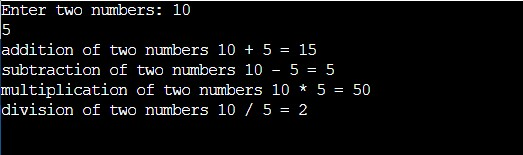In the above program, we have first initialized the required variable.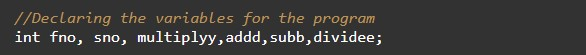• fno = it will hold the input number value from the user.
• sno = it will hold the input number value from the user.
• addd = it will hold the added value of the numbers.
• subb= it will hold the subtraction value of the numbers.

Taking Input numbers from the user.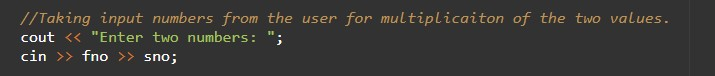Perform Addition, Subtraction, Multiplication, and Division of two numbers.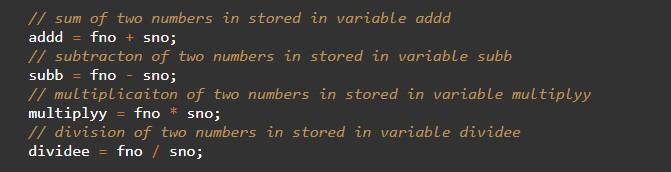Printing the output.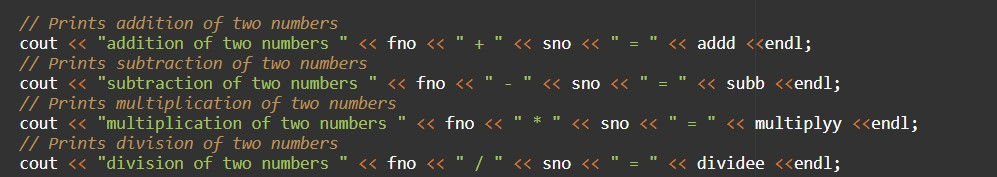In this tutorial we have learn about the C++ Program to Perform Addition, Subtraction, Multiplication and Division and its application with practical example. I hope you will like this tutorial.